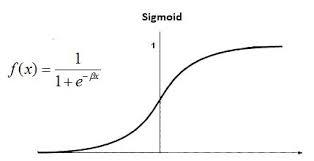• Last Updated : 02 Sep, 2020

Logistic regression is a classification algorithm used to find the probability of event success and event failure. It is used when the dependent variable is binary(0/1, True/False, Yes/No) in nature. It supports categorizing data into discrete classes by studying the relationship from a given set of labelled data. It learns a linear relationship from the given dataset and then introduces a non-linearity in the form of the Sigmoid function.Logistic regression is also known as Binomial logistics regression. It is based on sigmoid function where output is probability and input can be from -infinity to +infinity. Let’s discuss some advantages and disadvantages of Linear Regression.

Attention reader! Don’t stop learning now. Get hold of all the important Machine Learning Concepts with the Machine Learning Foundation Course at a student-friendly price and become industry ready.

My Personal Notes arrow_drop_up